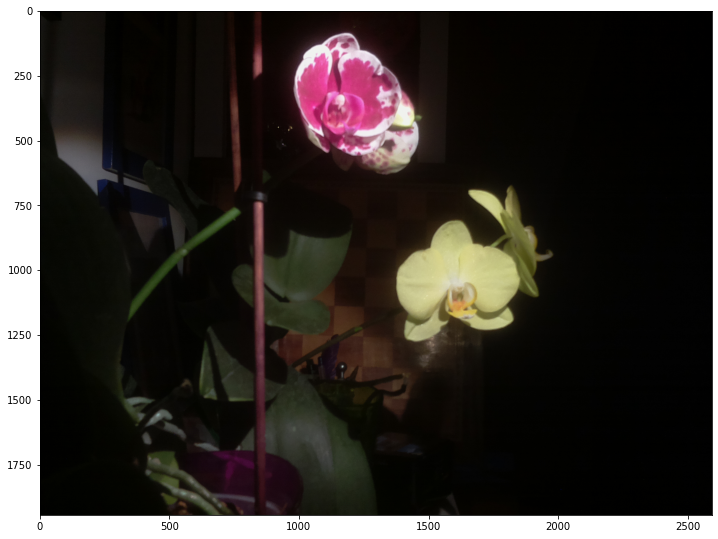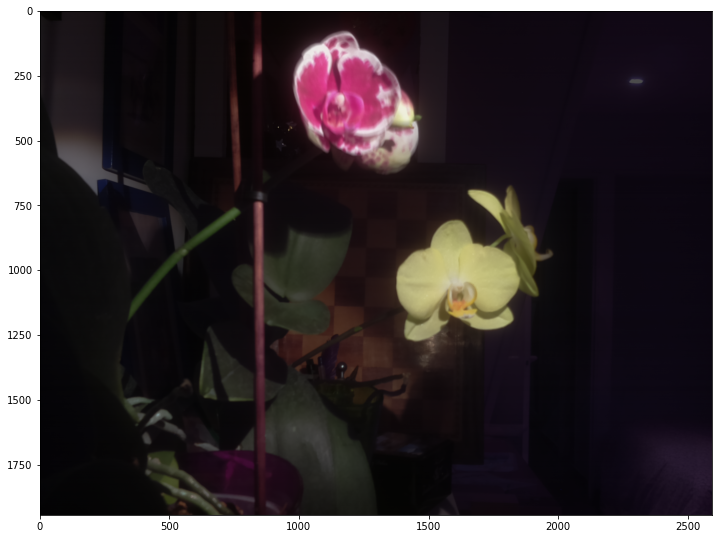# Time lapsing an orchid's flower

The goal here is to realize a time-lapse using a raspberry π and some python code and finally get this:(This was done over 10 days, with almost each day an irregular session the morning and one in the evening)

The code (and more) is also available @ https://github.com/laurentperrinet/TimeTeleScope

### data acquisition¶

This is well documented on the web and consisted in simply:

• setting up the raspberry π to use the camera
• create a startup script `startup.sh` (or clone this repo in `/home/pi` using `git clone https://github.com/laurentperrinet/TimeTeleScope)` consisting of the commands which take 2 successive frames at different exposures :
``````raspistill -rot 270 -ev -10 --metering spot -o /home/pi/Desktop/orchid/`date +%Y-%m-%d.%H:%M:%S`.jpg
raspistill -rot 270 -ev -8 --metering spot -o /home/pi/Desktop/orchid/`date +%Y-%m-%d.%H:%M:%S`.jpg``````
• run that script regularly (every ten minute) by adding the following line to the cron table (using `sudo crontab -e`):

``*/10 * * * * /home/pi/TimeTelescope/startup.sh``
• the π was placed next to the orchid flowers for more than one week.

The rest of this notebook transforms the sequence of frames into a movie.

## low-level utilities¶

In this first part, we:

• set up parameters,
• load images and display one,
• perform a low-level filtering of the good versus bad images
• todo: low-level re-alignment (seems not necessary as the camera did not move during the acquisition)
In :
```import numpy as np
import matplotlib
import matplotlib.pyplot as plt
phi = (np.sqrt(5)+1)/2
```
In :
```def init(args=[], date=None, DEBUG=1):
if date is None:
import datetime
# https://en.wikipedia.org/wiki/ISO_8601
date = datetime.datetime.now().date().isoformat()
tag = f'{date}_TimeTeleScope'

import argparse
# Training settings
parser = argparse.ArgumentParser(description='TimeTeleScope')
parser.add_argument('--tag', type=str, default=tag, help='unique ID to tag our results')
parser.add_argument('--date', type=str, default=date, help='date of the run')
parser.add_argument('--in-path', type=str, default='../orchid', help='Folder containing the raw images')
parser.add_argument('--cache-path', type=str, default='cache_path', help='Folder to store intermediate images')
parser.add_argument('--videos-path', type=str, default='videos', help='Folder to store the final video')
parser.add_argument('--figwidth', type=float, default=15, help='Width of figures')
parser.add_argument('--fps', type=float, default=12, help='FPS of output gif')
parser.add_argument('--verb', type=bool, default=0, help='to blah or not blah')
args = parser.parse_args(args=[])

return args
```
In :
```args = init()
args
```
Out:
`Namespace(tag='2021-02-20_TimeTeleScope', date='2021-02-20', in_path='../orchid', cache_path='cache_path', videos_path='videos', figwidth=15, ds=1, fps=12, verb=0)`
In :
```import os
def __init__(self, args):
# saving parameters in the class
self.args = args
if self.args.verb : print(f'Tagging our simulations with tag={self.args.tag}')

# creating folders
os.makedirs(self.args.cache_path, exist_ok=True)
os.makedirs(self.args.videos_path, exist_ok=True)
# setting up figure size
self.figsize = (self.args.figwidth, self.args.figwidth/phi)

# IMAGE
def glob(self, gex='*.jpg'):
"""
Globs images in a folder.

Images should have a jpg extension and
be in JPG format.

Returns a list of image filenames sorted alphabetically.
"""
fnames = []

import glob
# https://stackoverflow.com/questions/6773584/how-is-pythons-glob-glob-ordered
for fname in sorted(glob.glob(f'{self.args.in_path}/{gex}')):
fnames.append(fname)

return fnames

"""
Globs images in a folder.

Images should have a jpg extension and
be in JPG format.

Returns a list of images.
"""
imgs = {}
for fname in self.glob(gex=gex):
imgs[fname] = self.process(fname, do_preprend=False)
return imgs

def process(self, fname):
"""
Placeholder function for preprocessing images.

It simply consists here in normalizing the 8-bits raw images to be in the [0, 1] range.

"""
if self.args.verb >= 20 : print('img min max', img.min(), img.max())
return img/255.

"""

"""
import cv2
im = cv2.cvtColor(im, cv2.COLOR_BGR2RGB)

# resample by resizing image
nx, ny, three = im.shape
if self.args.ds > 1:
# CAREFUL ! for a strange reason openCV inverts H and W ??
im = cv2.resize(im, (ny//self.args.ds, nx//self.args.ds),
interpolation = cv2.INTER_AREA)
nx, ny, three = im.shape
assert(three==3)
return nx, ny, im
```

Instantiate the class:

In :
```ttl = TimeTeleScopeLoad(args)
```
In :
```fnames = ttl.glob()
```
In :
```print(f'{len(fnames)=}')
```
```len(fnames)=684
```

## histogram matching¶

The main processing step for setting up the time lapse is to make images have a similar lightning and color balance to avoid any flicker due to different lighting conditions.

The idea here is to separate two pieces of information: the palette of the image which corresponds to the different tones and colors within the scene and which depend of the lighting conditions versus the shape of the scene which is encoded in the position of the individual pixels. To avoid flickering, on efficient method is to tranfer the palette of a reference image to each image of the palette, and this is called technically histogram matching.

This equalization of images is performed with respect to a reference image, using the code from https://github.com/scikit-image/scikit-image/blob/master/skimage/exposure/histogram_matching.py#L22-L70 :

In :
```import numpy as np

from skimage.exposure import match_histograms

def histMatch(sourceImage, templateImage):
"""
Matches the histogram of sourceImage to the templateImage in order to fix lightness/exposure
of the sourceImage.
"""
return match_histograms(sourceImage, templateImage, multichannel=True)
```

Picking some image from the stack:

In :
```fname_reference = '../orchid/2021-02-14.22:10:07.jpg'
image_reference = ttl.process(fname_reference)
```
In :
```fig, ax = plt.subplots(figsize=ttl.figsize)
ax.imshow(image_reference, vmin=0, vmax=255);
```In :
```count = 1
for fname in fnames:
if '2021-02-14' in fname:
image_reference += ttl.process(fname)
count += 1
image_reference /= count

fig, ax = plt.subplots(figsize=ttl.figsize)
ax.imshow(image_reference)
plt.show();
print(image_reference.min(), image_reference.max())
``````0.0020988677161005243 0.9690140845070422
```

Picking another image from the stack, showing the same scene but with a different exposure:

In :
```fname_new = '../orchid/2021-02-14.17:30:07.jpg'
fig, ax = plt.subplots(figsize=ttl.figsize)
ax.imshow(ttl.process(fname_new));
```